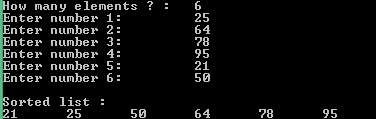## Friday, 27 March 2015

### Cpp Program for sorting elements using bubble sort

 This is a Cpp program that performs sorting of elements using bubble sort. Bubble sort is a technique where each element is compared with its adjacent element , if its adjacent element is smaller then swapping occurs, this continues until every element is checked.

See the animation below to understand,

"Bubble-sort-example-300px" by Swfung8 - Own work. Licensed under CC BY-SA 3.0 via Wikimedia Commons.

Download Code
PROGRAM :
```#include <iostream>

using namespace std;

int main()
{
int n, i, j, swap;
cout << "How many elements ? :\t";
cin >> n;
int array[n];
for (i = 0; i < n; i++)
{
cout << "Enter number " << i+1 << ": \t";
cin >> array[i];
}

for (i = 0 ; i < ( n - 1 ); i++)
{
for (j = 0 ; j < n - i - 1; j++)
{
if (array[j] > array[j+1])
{
swap       = array[j];
array[j]   = array[j+1];
array[j+1] = swap;
}
}
}
cout << "\nSorted list :\n";
for ( i = 0 ; i < n ; i++ )
cout << array[i] << "\t";
return 0;
}
```

OUTPUT  :Bubble Sort

Download Code